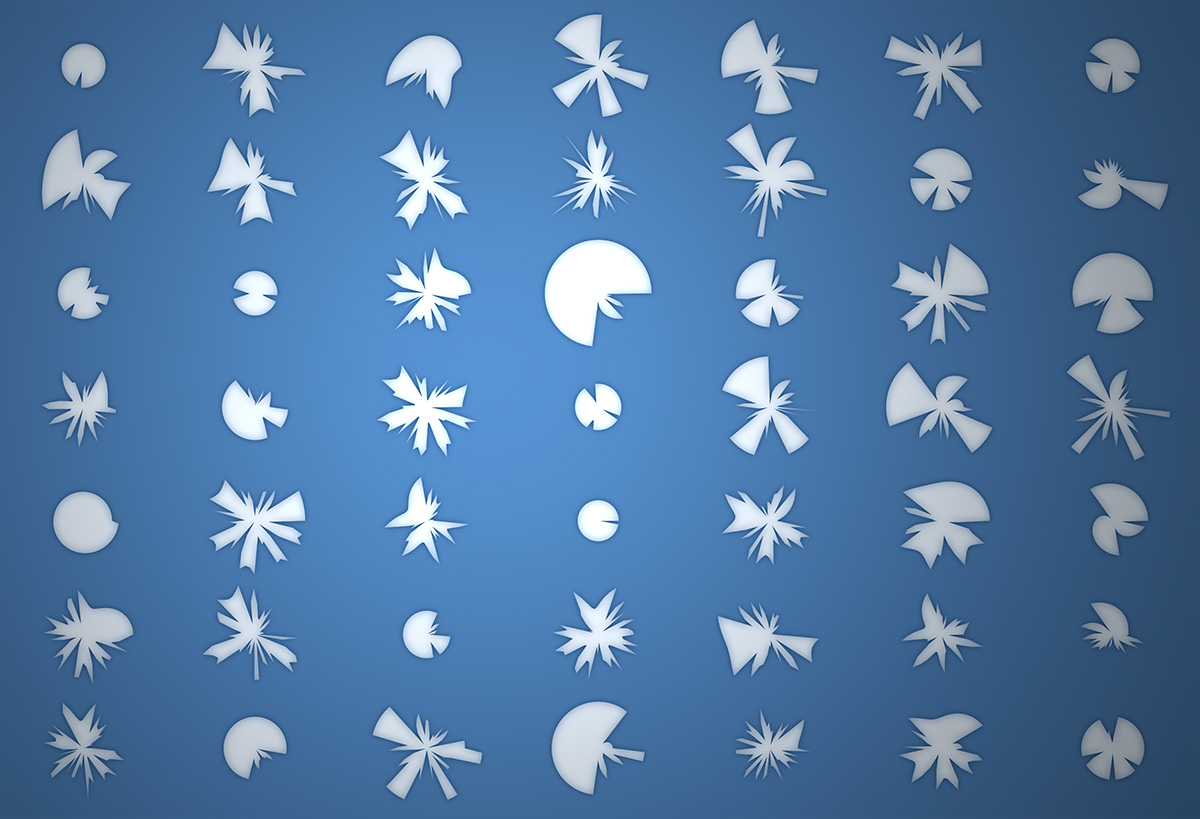# Category Archives: motion

In 2015 I created QuickShader… which just takes the boilerplate out of showing a shader in the browser. Here are a few examples:

Also posted in Graphics, Math, color, glsl, graphics algorithms, html5, javascript, pixel manipulation | Tagged , | Leave a comment

Awhile back I thought it would be interesting to add some quick fake lighting to a personal project of mine - that for lack of a better description is a windows management system.

Here is a screenshot of the windows management system with lighting turned on:Here is a video of me using the system:

I whipped up this prototype (don’t mind the jQuery)

There are really two keys that make this work. Getting the shadow in place and adjusting the gradient. All we really need is the angle and distance from a given `div` in relation to the “light”:

```1 2 3 4 5 6 7 8 9 10 11 12 13 let calcAng = function(x, y) { let lightPos = light.position() let dx = lightPos.left - x; let dy = lightPos.top - y; return -Math.atan2(dy, dx) / Math.PI * 180; };   let calcDist = function(x, y) { let lightPos = light.position() let dx = lightPos.left - x; let dy = lightPos.top - y; return Math.sqrt(dx * dx, dy * dy); };```

Standard `atan2` and the pythagorean theorem get us this. Once we have those - we can use them to set our gradient and shadow values:

```1 2 3 4 5 6 7 8 9 10 11 12 13 14 15 16 17 // warning (apparently this function is slightly speed coded) let calcShade = function(x, y) { let angle = calcAng(x, y); let dist = calcDist(x, y); let sx = dist * Math.cos(-angle * Math.PI / 180) * -1; let sy = dist * Math.sin(-angle * Math.PI / 180) * -1;   sx = Math.min(20, Math.max(sx, -20)); sy = Math.min(20, Math.max(sy, -20)); let blur = Math.min(100, dist); let hBlur = Math.min(50, blur) * 0.5; // consider distance in the eq? return { bg: `-webkit-linear-gradient(\${angle}deg, rgba(0, 0, 0, 0.2), rgba(255, 255, 255, 0.4) \${blur}%)`, shadow: `\${sx}px \${sy}px \${hBlur}px rgba(0, 0, 0, 0.15)` }; };```

There are more videos of the windows management system on my youtube channel. Here’s another from a much earlier version of the system.

Maybe I’ll post more about that in the future…

## Quick line in HTML and JavaScript

Usually if you want to draw lines in HTML you use canvas or SVG. Awhile back I wondered how I might do it without those. This is really just a proof of concept speed coded answer to that question:

This works by using a div with a border, rotating it and scaling it as needed so it fits between two arbitrary points.

This could be abstracted a bit more, but it works pretty well. I usually choose `setInterval` over `requestAnimationFrame` when prototyping - because I like to easily be able to change the speed of
framebased things like this. If I were to try and make this code more dynamic, I would probably switch out to `requestAnimationFrame`.

If you try and connect two lines together - you’ll notice some inaccuracy - a good argument for using SVG or canvas over something like this. That said, if you are connecting two elements using a single line, this inaccuracy would become irrelevant.

## Hermit Crab Curve as PRNG # (a deterministic pseudo-random number generator with contrast and smoothness - inspired by stochastic and organic randomness)

Around 2015 I had the idea for a PRNG that would clamp itself and have moments of “smoothness”. When I got around to trying to create such a thing, the result was something I jokingly called the “Hermit Crab Curve”. I also called it the “Shard Curve”.

The equation for the curve defines a radius in polar coordinates:Where a and d are paramters that control the detail of the curve. o is a rotation and offset value for the angle and s is a scalar. Note the use of rem. The resulting curve is much less interesting if a standard modulo is used in place of a remainder:The above variable values were found using this interactive version of the equation:

To illustrate how you might use this as a PRNG I created this fork of the above pen:

That, in combination with the information from my other article from yesterday… Should be enough to see what I mean.You can read the original description of the Hermit Crab Curve that I created using ArcType here:

If you end up using this for something interesting let me know. I’d love to see itAlso posted in Graphics, Math, functions, graphics algorithms, javascript, misc | Tagged , , | Leave a comment